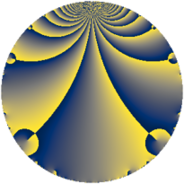# Properties

 Label 6930.2.a.byLevel $6930$ Weight $2$ Character orbit 6930.a Self dual yes Analytic conductor $55.336$ Analytic rank $0$ Dimension $2$ CM no Inner twists $1$

# Related objects

## Newspace parameters

 Level: $$N$$ $$=$$ $$6930 = 2 \cdot 3^{2} \cdot 5 \cdot 7 \cdot 11$$ Weight: $$k$$ $$=$$ $$2$$ Character orbit: $$[\chi]$$ $$=$$ 6930.a (trivial)

## Newform invariants

 Self dual: yes Analytic conductor: $$55.3363286007$$ Analytic rank: $$0$$ Dimension: $$2$$ Coefficient field: $$\Q(\sqrt{33})$$ Defining polynomial: $$x^{2} - x - 8$$ Coefficient ring: $$\Z[a_1, \ldots, a_{13}]$$ Coefficient ring index: $$2$$ Twist minimal: no (minimal twist has level 2310) Fricke sign: $$-1$$ Sato-Tate group: $\mathrm{SU}(2)$

## $q$-expansion

Coefficients of the $$q$$-expansion are expressed in terms of $$\beta = \sqrt{33}$$. We also show the integral $$q$$-expansion of the trace form.

 $$f(q)$$ $$=$$ $$q + q^{2} + q^{4} - q^{5} - q^{7} + q^{8} +O(q^{10})$$ $$q + q^{2} + q^{4} - q^{5} - q^{7} + q^{8} - q^{10} + q^{11} + ( 1 + \beta ) q^{13} - q^{14} + q^{16} + ( 1 + \beta ) q^{17} - q^{20} + q^{22} + ( 1 - \beta ) q^{23} + q^{25} + ( 1 + \beta ) q^{26} - q^{28} -2 q^{29} + q^{32} + ( 1 + \beta ) q^{34} + q^{35} + ( 3 - \beta ) q^{37} - q^{40} -6 q^{41} + 4 q^{43} + q^{44} + ( 1 - \beta ) q^{46} + 4 q^{47} + q^{49} + q^{50} + ( 1 + \beta ) q^{52} + 6 q^{53} - q^{55} - q^{56} -2 q^{58} -4 q^{59} + 6 q^{61} + q^{64} + ( -1 - \beta ) q^{65} + ( -7 - \beta ) q^{67} + ( 1 + \beta ) q^{68} + q^{70} + 6 q^{73} + ( 3 - \beta ) q^{74} - q^{77} + 12 q^{79} - q^{80} -6 q^{82} + 4 q^{83} + ( -1 - \beta ) q^{85} + 4 q^{86} + q^{88} + ( -1 - \beta ) q^{89} + ( -1 - \beta ) q^{91} + ( 1 - \beta ) q^{92} + 4 q^{94} + ( 4 + 2 \beta ) q^{97} + q^{98} +O(q^{100})$$ $$\operatorname{Tr}(f)(q)$$ $$=$$ $$2 q + 2 q^{2} + 2 q^{4} - 2 q^{5} - 2 q^{7} + 2 q^{8} + O(q^{10})$$ $$2 q + 2 q^{2} + 2 q^{4} - 2 q^{5} - 2 q^{7} + 2 q^{8} - 2 q^{10} + 2 q^{11} + 2 q^{13} - 2 q^{14} + 2 q^{16} + 2 q^{17} - 2 q^{20} + 2 q^{22} + 2 q^{23} + 2 q^{25} + 2 q^{26} - 2 q^{28} - 4 q^{29} + 2 q^{32} + 2 q^{34} + 2 q^{35} + 6 q^{37} - 2 q^{40} - 12 q^{41} + 8 q^{43} + 2 q^{44} + 2 q^{46} + 8 q^{47} + 2 q^{49} + 2 q^{50} + 2 q^{52} + 12 q^{53} - 2 q^{55} - 2 q^{56} - 4 q^{58} - 8 q^{59} + 12 q^{61} + 2 q^{64} - 2 q^{65} - 14 q^{67} + 2 q^{68} + 2 q^{70} + 12 q^{73} + 6 q^{74} - 2 q^{77} + 24 q^{79} - 2 q^{80} - 12 q^{82} + 8 q^{83} - 2 q^{85} + 8 q^{86} + 2 q^{88} - 2 q^{89} - 2 q^{91} + 2 q^{92} + 8 q^{94} + 8 q^{97} + 2 q^{98} + O(q^{100})$$

## Embeddings

For each embedding $$\iota_m$$ of the coefficient field, the values $$\iota_m(a_n)$$ are shown below.

For more information on an embedded modular form you can click on its label.

Label $$\iota_m(\nu)$$ $$a_{2}$$ $$a_{3}$$ $$a_{4}$$ $$a_{5}$$ $$a_{6}$$ $$a_{7}$$ $$a_{8}$$ $$a_{9}$$ $$a_{10}$$
1.1
 −2.37228 3.37228
1.00000 0 1.00000 −1.00000 0 −1.00000 1.00000 0 −1.00000
1.2 1.00000 0 1.00000 −1.00000 0 −1.00000 1.00000 0 −1.00000
 $$n$$: e.g. 2-40 or 990-1000 Significant digits: Format: Complex embeddings Normalized embeddings Satake parameters Satake angles

## Atkin-Lehner signs

$$p$$ Sign
$$2$$ $$-1$$
$$3$$ $$-1$$
$$5$$ $$1$$
$$7$$ $$1$$
$$11$$ $$-1$$

## Inner twists

This newform does not admit any (nontrivial) inner twists.

## Twists

By twisting character orbit
Char Parity Ord Mult Type Twist Min Dim
1.a even 1 1 trivial 6930.2.a.by 2
3.b odd 2 1 2310.2.a.x 2

By twisted newform orbit
Twist Min Dim Char Parity Ord Mult Type
2310.2.a.x 2 3.b odd 2 1
6930.2.a.by 2 1.a even 1 1 trivial

## Hecke kernels

This newform subspace can be constructed as the intersection of the kernels of the following linear operators acting on $$S_{2}^{\mathrm{new}}(\Gamma_0(6930))$$:

 $$T_{13}^{2} - 2 T_{13} - 32$$ $$T_{17}^{2} - 2 T_{17} - 32$$ $$T_{19}$$ $$T_{23}^{2} - 2 T_{23} - 32$$ $$T_{29} + 2$$ $$T_{31}$$

## Hecke characteristic polynomials

$p$ $F_p(T)$
$2$ $$( -1 + T )^{2}$$
$3$ $$T^{2}$$
$5$ $$( 1 + T )^{2}$$
$7$ $$( 1 + T )^{2}$$
$11$ $$( -1 + T )^{2}$$
$13$ $$-32 - 2 T + T^{2}$$
$17$ $$-32 - 2 T + T^{2}$$
$19$ $$T^{2}$$
$23$ $$-32 - 2 T + T^{2}$$
$29$ $$( 2 + T )^{2}$$
$31$ $$T^{2}$$
$37$ $$-24 - 6 T + T^{2}$$
$41$ $$( 6 + T )^{2}$$
$43$ $$( -4 + T )^{2}$$
$47$ $$( -4 + T )^{2}$$
$53$ $$( -6 + T )^{2}$$
$59$ $$( 4 + T )^{2}$$
$61$ $$( -6 + T )^{2}$$
$67$ $$16 + 14 T + T^{2}$$
$71$ $$T^{2}$$
$73$ $$( -6 + T )^{2}$$
$79$ $$( -12 + T )^{2}$$
$83$ $$( -4 + T )^{2}$$
$89$ $$-32 + 2 T + T^{2}$$
$97$ $$-116 - 8 T + T^{2}$$